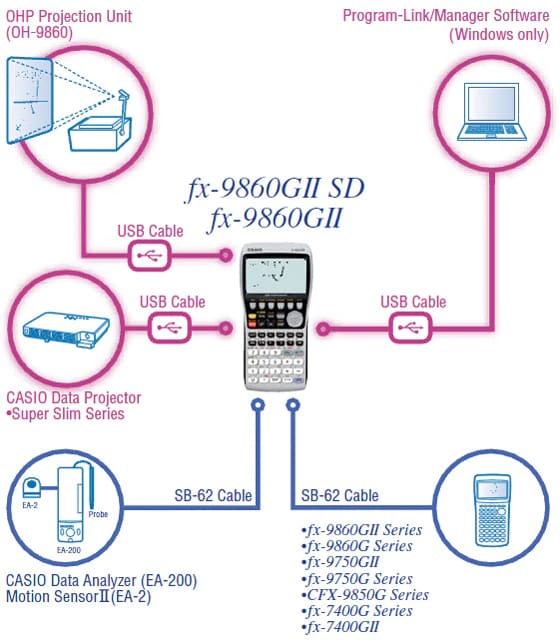# fx-9860GII | CASIO

Search

Natural textbook display
Graphing Calculators

# fx-9860GII

My GShock

## Features## User-friendly Interface

Rectangular coordinate graphing, Polar coordinate graphing

Integration graph

Parametric function graphing, Inequality graphing

Trace, Zoom (box zoom, zoom in, zoom out, auto zoom)

Table and Graph

Dual Graph (table and graph, graph and graph)

Sketch (tangent line, normal line, inverse function)

Solve (root, minimum, maximum, intersection, integration)

Dynamic graph

Conic section graph

Recursion graph

List-based one-variable and two-variable statistical analysis

Statistical regression calculations

Statistical plot (scatter plot, xyLine, normal probability plot, histogram, box plot)

Statistical regression graphs (linear, med-med, quadratic, cubic, quartic, logarithmic, exponential, power, sinusoidal, logistic regression)

Advanced statistical calculations: tests (Z-test, t-test, Chi square test, F-test, ANOVA), intervals (Z-interval, t-interval), distributions

Pie chart

Bar graph

Power functions (square root, cubic root, square, power, radical root)

GCD/LCM

Coordinate conversion (Pol, Rec)

Combination/Permutation (nCr, nPr)

Factorial, Inverse, random numbers, Fractions

Logical operations

Matrix calculations

Complex number calculations

Base-n calculations/conversions

List data calculations

Metric Conversion

Natural format equation output

Calculation history

Numeric equation solver, simultaneous equations, polynomial equations

Financial calculations

Programming

Data communication

User memory: 62,000 bytes, User Storage memory: 1.5 M bytes

## Hardware Features

#### High-resolution LCD

The large 64 × 128-dot display of the fx-9860GII Series high-resolution LCD produces formulas, graphs and graphics that are sharper, clearer, and easier to read.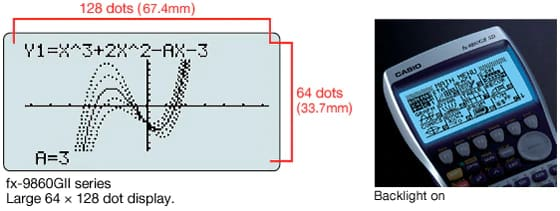#### High-speed CPU

A high-performance, high-speed CPU gives fx-9860GII Series calculators processing speeds that are three to five time faster than other brand calculators in their class. Processes and plots encountered in complex calculations and graphics are handled with ease, for enhanced operational efficiency and learning as well.

#### Out-of-the-box USB Operations

A USB cable, unit-to-unit cable and Program-Link Software all are included with the calculator, so high-speed data communication with a computer as well as unit-to-unit data and program transfers can be performed virtually out of the box.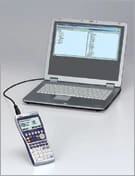## Natural textbook display!

CASIO's original "Natural Expression Input Display" and "Natural Expression Output Display" make it possible to display fractions, exponents, logarithms, powers, and square roots just as they are written in the textbook. The result is enhanced student comprehension and improved math class efficiency.

#### Natural Input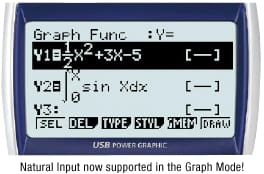#### Polynomial equation result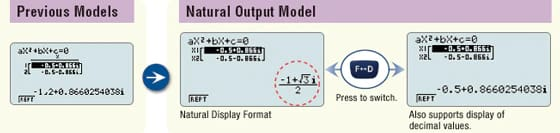#### Result of expression with Pi symbol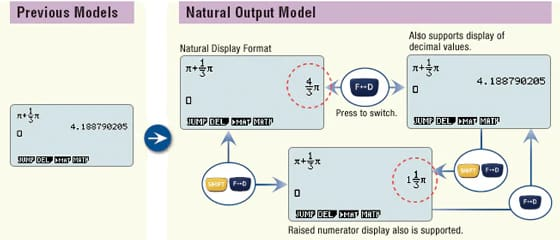## eActivity

fx-9860GII Series calculators come with the same eActivity capabilities that originally appeared on the ClassPad 330. Now teachers as well as students can create their own problems and study materials. Students get the opportunity to learn at their own pace for more efficient study both at school and at home. eActivity is a great motivator for learning and understanding.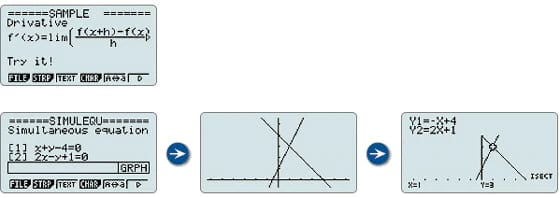## Built-in Software

A multi-function spreadsheet with built-in graphing capabilities is a valuable tool for table calculation lesson exercises.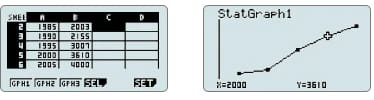#### E-CON2

E-CON2 provides total control over the optional EA-200 Data Analyzer. It makes it possible to measure changes in temperature, sound, or speed using the EA-200 without any troublesome settings or program input.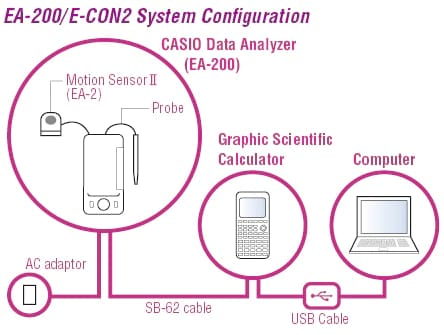#### Pre-installed Software

Pre-installed add-in software comes installed on the calculator when you purchase it.
You can use such software as-is, or you can delete it to free up memory.

#### Geometry

Geometry add-in software is designed to make learning geometry fun.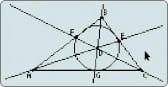#### Physium

The Physium add-in provides instant access to the periodic table of elements, whose data can be used in calculations. Often-use elements and atomic symbols can be stored for quick and easy recall whenever you need them.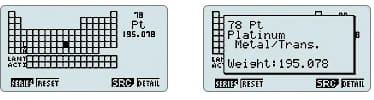## Other Features

#### Probability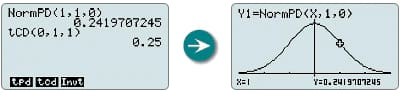Normal distribution, student's t-distribution, and other often-used statistical calculations are provided in function format for easier practical application.

#### Inequality Graphing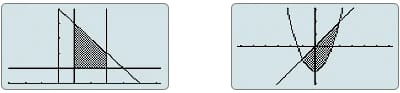New support for graphing the inequality of an x=Constant graph and x=f ( y) graph allows study of the area for which the x-range is defined.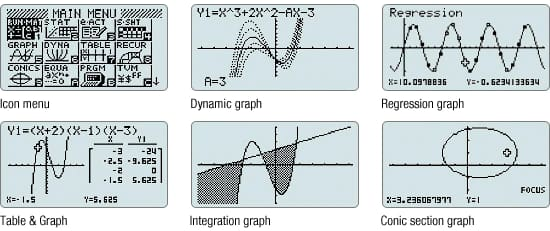#### List-based Statistics

Store a list of values in memory for use when performing function and statistical calculations, when drawing graphs, or when generating tables of numeric values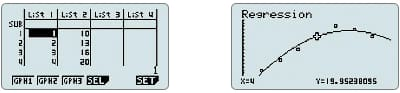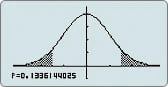Perform tests, confidence interval, probability distribution, and other calculations and graphing.1-sample t-test graph

#### Financial Calculations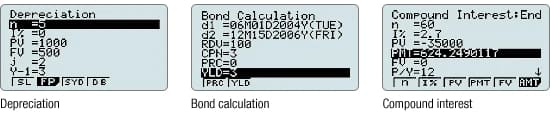Depreciation

Bond calculation

Simple interest

Compound interest

Investment appraisal (cash flow)

Amortization

Interest rate conversion (annual percentage rate and effective interest rate)

Cost, selling price, or margin

Day or date calculations

#### Peripherals